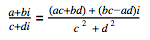# IMDIV

Returns one complex number divided by another.

### Sample Usage

`IMDIV("11+16i", "3+2i")`

`IMDIV("4+2j", 2)`

### Syntax

`IMDIV(dividend, divisor)`

• `dividend` - The complex number to be divided.
• `divisor` - The complex number to divide by.

### Notes

• The division between two complex numbers is defined as follows:
•• You can divide two complex numbers only if they have the same suffix (i or j). For example, you can't do `IMDIV("4+3i", "1+2j")`.

### Examples

1 A B
2 Formula Result
3 `=IMDIV("11+16i", "3+2i")` 5+2i
4 `=IMDIV("4+2j", 2)` 2+j
5 `=IMDIV(COMPLEX(11, 16), COMPLEX(3, 2))` 5+2i